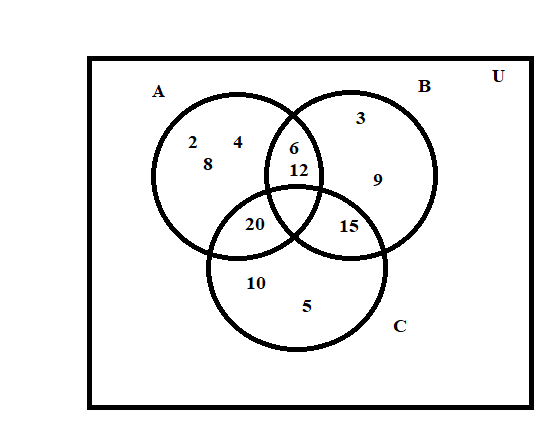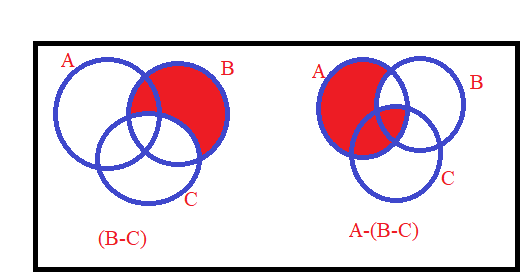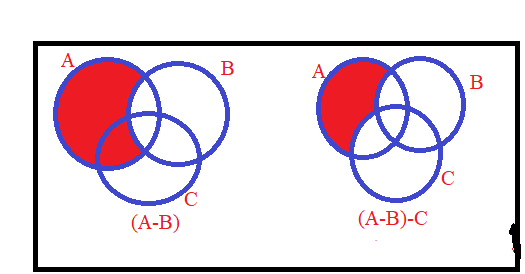June 28, 2022

# Set Theory(NCERT Exemplar )

Here we have given the solutions of  some important questions of chapter 1 (Set Theory) of NCERT Exemplar book .

1. Write the following sets in the roaster  form

(i)

(ii)

(iii)

Sol. (i) On solving the equation      , we get

Thus     .

(ii)       gives    which implies

Thus

(iii) Since every prime no. has  only two factors 1 and number itself. This implies

2.  Write the following sets in the roaster  form:

(i)

(ii)

(iii)

Sol. (i) On solving   , we get     ,  which implies  either or  .

Thus

(ii)                implies

It gives  .

Thus .

(iii)   We have

This implies      .

Therefore  .

Q3. If . Write in the roaster form.

sol. .

Since   is prime number , it has only two factors 1 and ( ) and  the factors of are 1,2,22,23,…, .   Therefore .

Q4. ,    and are subsets of Universal Set .  If  and is the set of all whole numbers, draw a Venn diagram showing the relation of and .

Sol. Here

Therefore , and .

Hence, the Venn diagram showing relation of given sets is:5. Determine whether  the statement given below   is true or false. Justify your answer.

For all sets , and ,

Solution:  Let ,

then  . This implies               …………..(i)

Now , this implies                      …………..(ii)

From (i) and (ii) , it is clear that .

We can also show that the given expression is false by using Venn DiagramsNow, from the Venn diagrams,  it is clear that .#### Bina singh

View all posts by Bina singh →
IBPS Clerk Exam Date 2022 Out SSC MTS Admit Card 2022 Government Exam Calendar July 2022 CBSE Class 11 Mathematics Revised Syllabus 2022-2023 UGC NET 2022 Exam Date